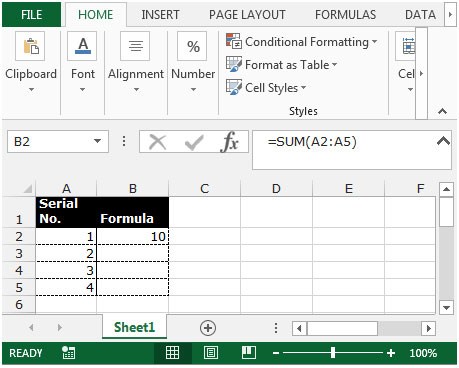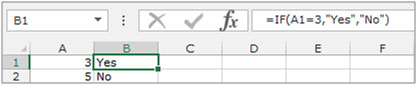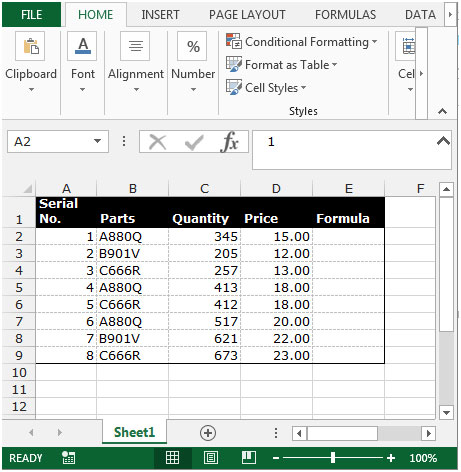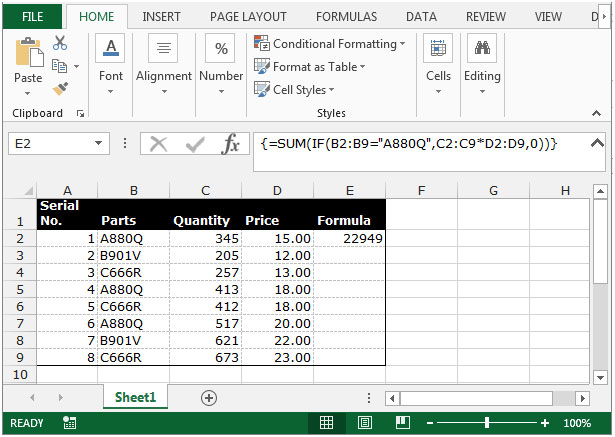# Sum total stock value for any part in Excel 2010

To calculate the total stock value for any part, we use the SUM function along with the IF function in Excel 2010.

SUM: - This function is used to add / sum all the numbers in a range of cells.

Syntax of SUM function: -=SUM(number1, number2,……)

For example:Cells A2:A5 contain numbers.We need to add all the numbers and show the result in cell B2.

• Select the cell B2.
• Write the formula =SUM(A2:A5)
• Press Enter on the keyboard.
• The function will add all the numbers.IF: - Checks whether a condition is met and returns one value if True and another value if False.

Syntax of “IF” function =IF(logical test,[value_if_true],[value_if_false])

First the formula will perform a logical test and return one value if the logical test is true and another value if the test is false.

For example:Cells A2 and A3 contain the numbers 3 and 5. If the number is 3, then the formula should display “Yes”, else “No”.

=IF (A1=3,"Yes","No")Let’s take an example to understand how we can calculate the total stock value for any part.

We have data showing the parts and their respective quantity sold with the amounts. We need to return the total sold amount for product A880Q.To calculate the total stock value follow below given steps.

• Select the cell E2 and write the formula.
• =SUM(IF(B2:B9="A880Q",C2:C9*D2:D9,0))
• Press Ctrl+Shift+Enter on your keyboard.
• The function will return the total stock value for the product A880Q.Note: This is an array function.After entering the formula in the cell press F2 and then press Ctrl+Shift+Enter on your keyboard.

This is the way we can calculate the total stock value by using the SUM function along with the IF function in Microsoft Excel.

## Users are saying about us...

1.A superb little trick that I had forgotten how to do - one of those useful tips that saves loads of file space and makes it easier to understand the calculation.

2."I totally follow the instructions above but my results are
not as same as showed. The Part do not sum for all item, but sum only for the certain row.
What shold I do, I am assigned to prepare a worksheet which have the result of sum of each product like this.

3.You can autosum with shorcut key alt+= in M.S. Excel

4."Are you using the data in the example above?

If so, please can you post back with EXACTLY what you see in the formula bar in Excel (should start with an open curly bracket { as above), and what result you are getting. "

Terms and Conditions of use

The applications/code on this site are distributed as is and without warranties or liability. In no event shall the owner of the copyrights, or the authors of the applications/code be liable for any loss of profit, any problems or any damage resulting from the use or evaluation of the applications/code.